Anzeige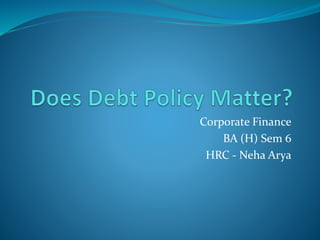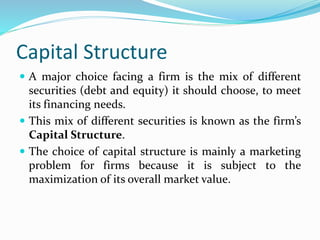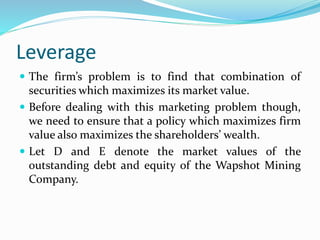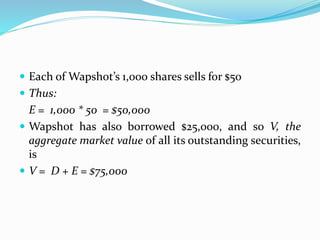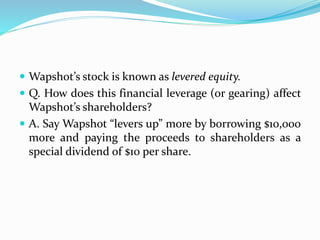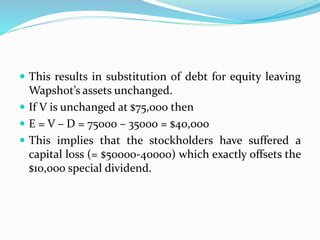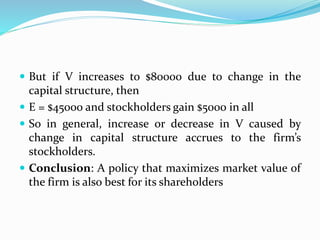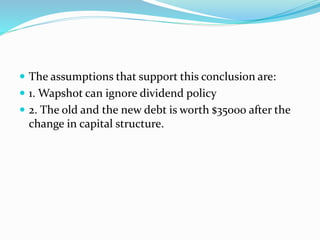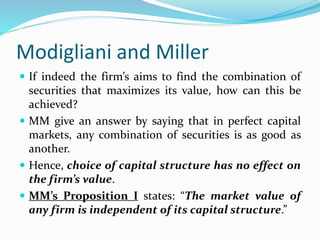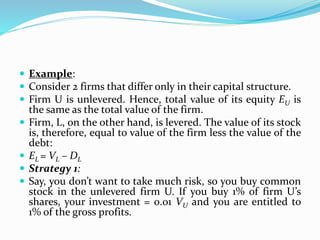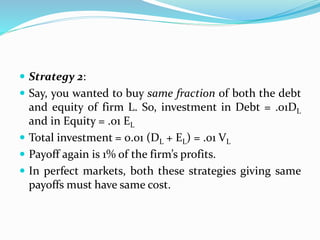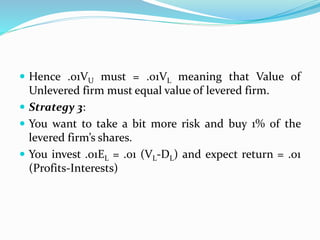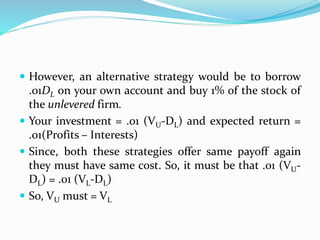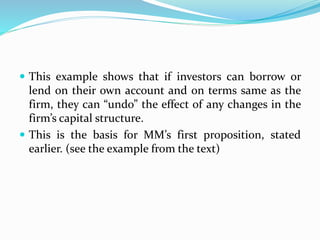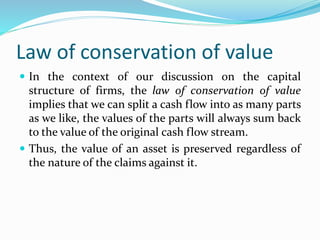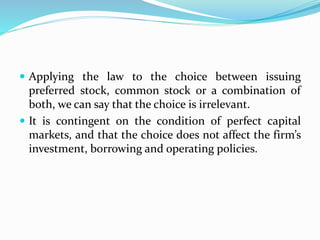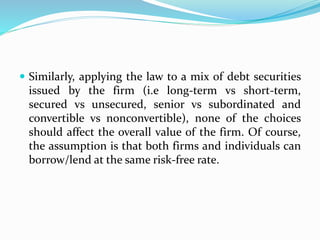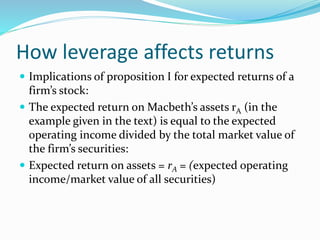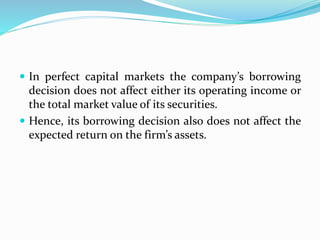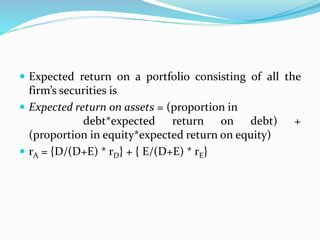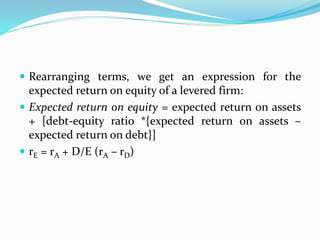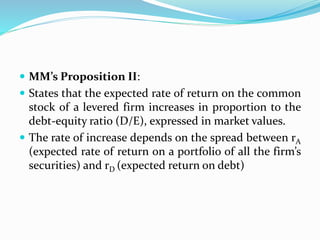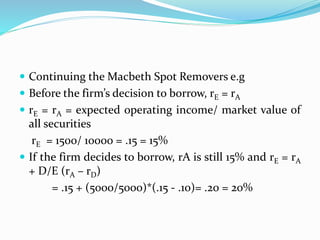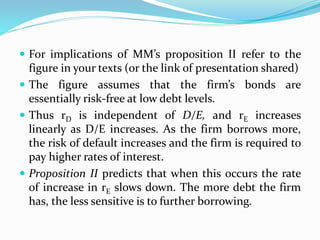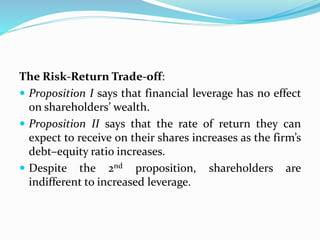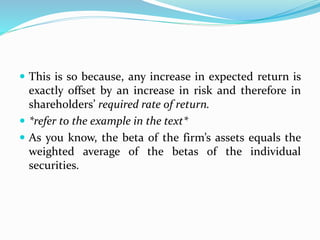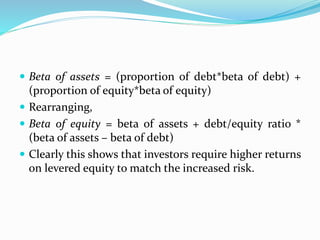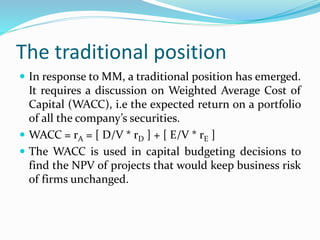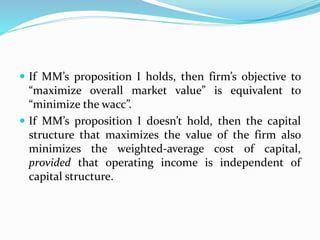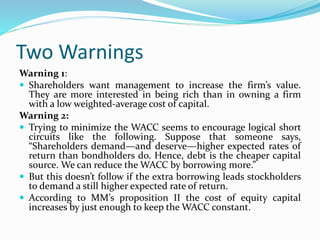1 von 31
Anzeige

### Does_Debt_Policy_Matter.pptx

1. Corporate Finance BA (H) Sem 6 HRC - Neha Arya
2. Capital Structure  A major choice facing a firm is the mix of different securities (debt and equity) it should choose, to meet its financing needs.  This mix of different securities is known as the firm’s Capital Structure.  The choice of capital structure is mainly a marketing problem for firms because it is subject to the maximization of its overall market value.
3. Leverage  The firm’s problem is to find that combination of securities which maximizes its market value.  Before dealing with this marketing problem though, we need to ensure that a policy which maximizes firm value also maximizes the shareholders’ wealth.  Let D and E denote the market values of the outstanding debt and equity of the Wapshot Mining Company.
4.  Each of Wapshot’s 1,000 shares sells for \$50  Thus: E = 1,000 * 50 = \$50,000  Wapshot has also borrowed \$25,000, and so V, the aggregate market value of all its outstanding securities, is  V = D + E = \$75,000
5.  Wapshot’s stock is known as levered equity.  Q. How does this financial leverage (or gearing) affect Wapshot’s shareholders?  A. Say Wapshot “levers up” more by borrowing \$10,000 more and paying the proceeds to shareholders as a special dividend of \$10 per share.
6.  This results in substitution of debt for equity leaving Wapshot’s assets unchanged.  If V is unchanged at \$75,000 then  E = V – D = 75000 – 35000 = \$40,000  This implies that the stockholders have suffered a capital loss (= \$50000-40000) which exactly offsets the \$10,000 special dividend.
7.  But if V increases to \$80000 due to change in the capital structure, then  E = \$45000 and stockholders gain \$5000 in all  So in general, increase or decrease in V caused by change in capital structure accrues to the firm’s stockholders.  Conclusion: A policy that maximizes market value of the firm is also best for its shareholders
8.  The assumptions that support this conclusion are:  1. Wapshot can ignore dividend policy  2. The old and the new debt is worth \$35000 after the change in capital structure.
9. Modigliani and Miller  If indeed the firm’s aims to find the combination of securities that maximizes its value, how can this be achieved?  MM give an answer by saying that in perfect capital markets, any combination of securities is as good as another.  Hence, choice of capital structure has no effect on the firm’s value.  MM’s Proposition I states: “The market value of any firm is independent of its capital structure.”
10.  Example:  Consider 2 firms that differ only in their capital structure.  Firm U is unlevered. Hence, total value of its equity EU is the same as the total value of the firm.  Firm, L, on the other hand, is levered. The value of its stock is, therefore, equal to value of the firm less the value of the debt:  EL = VL – DL  Strategy 1:  Say, you don’t want to take much risk, so you buy common stock in the unlevered firm U. If you buy 1% of firm U’s shares, your investment = 0.01 VU and you are entitled to 1% of the gross profits.
11.  Strategy 2:  Say, you wanted to buy same fraction of both the debt and equity of firm L. So, investment in Debt = .01DL and in Equity = .01 EL  Total investment = 0.01 (DL + EL) = .01 VL  Payoff again is 1% of the firm’s profits.  In perfect markets, both these strategies giving same payoffs must have same cost.
12.  Hence .01VU must = .01VL meaning that Value of Unlevered firm must equal value of levered firm.  Strategy 3:  You want to take a bit more risk and buy 1% of the levered firm’s shares.  You invest .01EL = .01 (VL-DL) and expect return = .01 (Profits-Interests)
13.  However, an alternative strategy would be to borrow .01DL on your own account and buy 1% of the stock of the unlevered firm.  Your investment = .01 (VU-DL) and expected return = .01(Profits – Interests)  Since, both these strategies offer same payoff again they must have same cost. So, it must be that .01 (VU- DL) = .01 (VL-DL)  So, VU must = VL
14.  This example shows that if investors can borrow or lend on their own account and on terms same as the firm, they can “undo” the effect of any changes in the firm’s capital structure.  This is the basis for MM’s first proposition, stated earlier. (see the example from the text)
15. Law of conservation of value  In the context of our discussion on the capital structure of firms, the law of conservation of value implies that we can split a cash flow into as many parts as we like, the values of the parts will always sum back to the value of the original cash flow stream.  Thus, the value of an asset is preserved regardless of the nature of the claims against it.
16.  Applying the law to the choice between issuing preferred stock, common stock or a combination of both, we can say that the choice is irrelevant.  It is contingent on the condition of perfect capital markets, and that the choice does not affect the firm’s investment, borrowing and operating policies.
17.  Similarly, applying the law to a mix of debt securities issued by the firm (i.e long-term vs short-term, secured vs unsecured, senior vs subordinated and convertible vs nonconvertible), none of the choices should affect the overall value of the firm. Of course, the assumption is that both firms and individuals can borrow/lend at the same risk-free rate.
18. How leverage affects returns  Implications of proposition I for expected returns of a firm’s stock:  The expected return on Macbeth’s assets rA (in the example given in the text) is equal to the expected operating income divided by the total market value of the firm’s securities:  Expected return on assets = rA = (expected operating income/market value of all securities)
19.  In perfect capital markets the company’s borrowing decision does not affect either its operating income or the total market value of its securities.  Hence, its borrowing decision also does not affect the expected return on the firm’s assets.
20.  Expected return on a portfolio consisting of all the firm’s securities is  Expected return on assets = (proportion in debt*expected return on debt) + (proportion in equity*expected return on equity)  rA = {D/(D+E) * rD} + { E/(D+E) * rE}
21.  Rearranging terms, we get an expression for the expected return on equity of a levered firm:  Expected return on equity = expected return on assets + [debt-equity ratio *{expected return on assets – expected return on debt}]  rE = rA + D/E (rA – rD)
22.  MM’s Proposition II:  States that the expected rate of return on the common stock of a levered firm increases in proportion to the debt-equity ratio (D/E), expressed in market values.  The rate of increase depends on the spread between rA (expected rate of return on a portfolio of all the firm’s securities) and rD (expected return on debt)
23.  Continuing the Macbeth Spot Removers e.g  Before the firm’s decision to borrow, rE = rA  rE = rA = expected operating income/ market value of all securities rE = 1500/ 10000 = .15 = 15%  If the firm decides to borrow, rA is still 15% and rE = rA + D/E (rA – rD) = .15 + (5000/5000)*(.15 - .10)= .20 = 20%
24.  For implications of MM’s proposition II refer to the figure in your texts (or the link of presentation shared)  The figure assumes that the firm’s bonds are essentially risk-free at low debt levels.  Thus rD is independent of D/E, and rE increases linearly as D/E increases. As the firm borrows more, the risk of default increases and the firm is required to pay higher rates of interest.  Proposition II predicts that when this occurs the rate of increase in rE slows down. The more debt the firm has, the less sensitive is to further borrowing.
25. The Risk-Return Trade-off:  Proposition I says that financial leverage has no effect on shareholders’ wealth.  Proposition II says that the rate of return they can expect to receive on their shares increases as the firm’s debt–equity ratio increases.  Despite the 2nd proposition, shareholders are indifferent to increased leverage.
26.  This is so because, any increase in expected return is exactly offset by an increase in risk and therefore in shareholders’ required rate of return.  *refer to the example in the text*  As you know, the beta of the firm’s assets equals the weighted average of the betas of the individual securities.
27.  Beta of assets = (proportion of debt*beta of debt) + (proportion of equity*beta of equity)  Rearranging,  Beta of equity = beta of assets + debt/equity ratio * (beta of assets – beta of debt)  Clearly this shows that investors require higher returns on levered equity to match the increased risk.
28. The traditional position  In response to MM, a traditional position has emerged. It requires a discussion on Weighted Average Cost of Capital (WACC), i.e the expected return on a portfolio of all the company’s securities.  WACC = rA = [ D/V * rD ] + [ E/V * rE ]  The WACC is used in capital budgeting decisions to find the NPV of projects that would keep business risk of firms unchanged.
29.  If MM’s proposition I holds, then firm’s objective to “maximize overall market value” is equivalent to “minimize the wacc”.  If MM’s proposition I doesn’t hold, then the capital structure that maximizes the value of the firm also minimizes the weighted-average cost of capital, provided that operating income is independent of capital structure.
30. Two Warnings Warning 1:  Shareholders want management to increase the firm’s value. They are more interested in being rich than in owning a firm with a low weighted-average cost of capital. Warning 2:  Trying to minimize the WACC seems to encourage logical short circuits like the following. Suppose that someone says, “Shareholders demand—and deserve—higher expected rates of return than bondholders do. Hence, debt is the cheaper capital source. We can reduce the WACC by borrowing more.”  But this doesn’t follow if the extra borrowing leads stockholders to demand a still higher expected rate of return.  According to MM’s proposition II the cost of equity capital increases by just enough to keep the WACC constant.
Anzeige